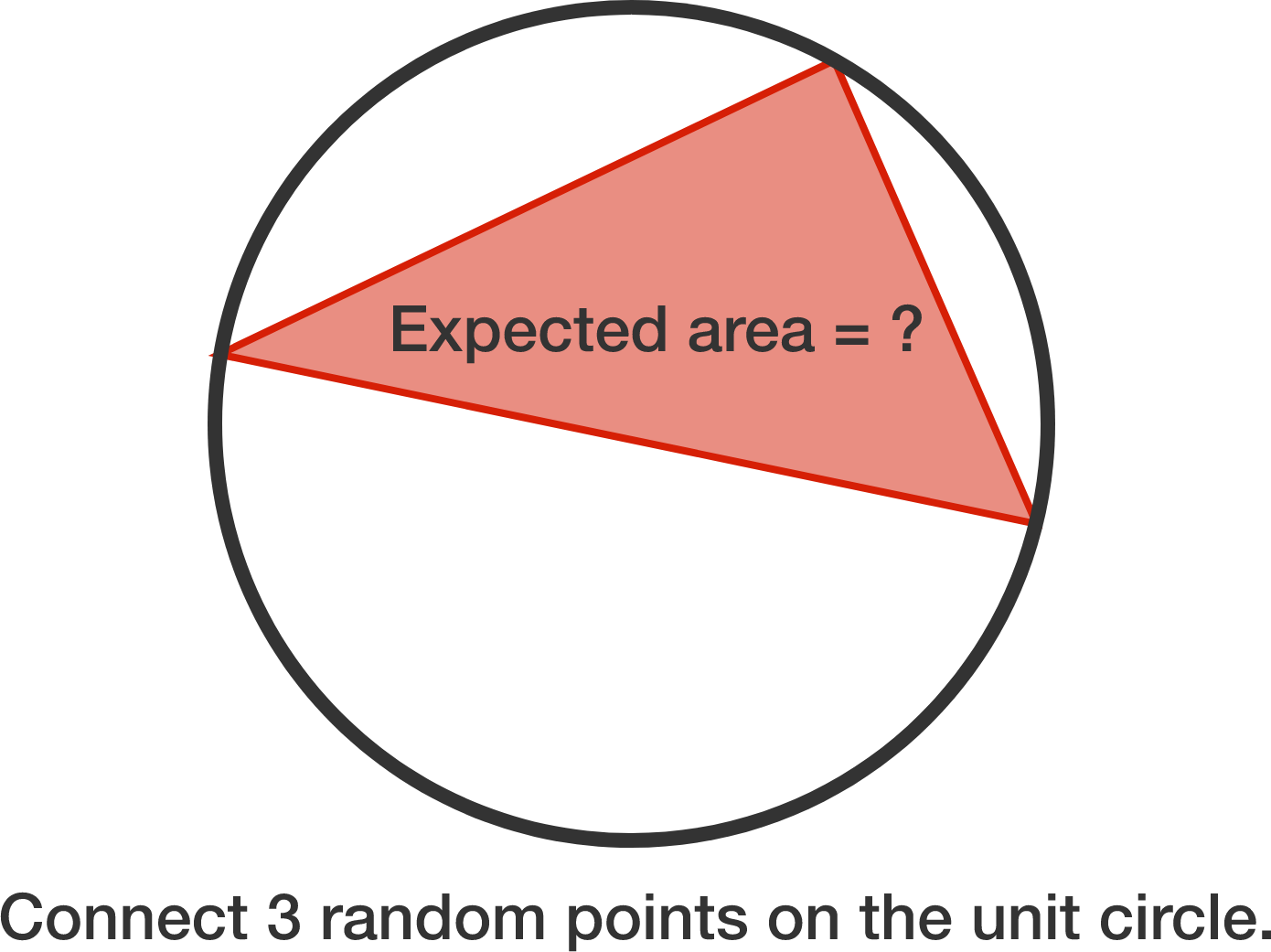# Expected value of triangle area

Calculus Level 3

Three points are chosen at random on the circumference of a circle with radius 1. Find the expected value, $v$, of the area of the triangle formed by the three points.

If $v = \dfrac{a}{b \pi}$, where $a$ and $b$ are coprime positive integers, submit $a+b$.×